Want to share your content on R-bloggers? click here if you have a blog, or here if you don't.## The problem

Consider a case where we have a bag of marbles of size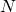. The bag consists ofblack marbles andwhite marbles. In this examplemeaning there is less black marbles than white. Each black marble has a number between 1 andis paired with a white marble which are labeled between 1 and. From the bag of marbles we take a sample of size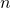. The problem is, what is the probability of selecting at least 1 pair of marbles i.e. at least 1 black and white marble with the same label?

For this example consider the following values:

•marbles
•marbles drawn from the bag
•black marbles in the bag

This can be broken into two parts:

1. The probability of selecting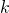black marbles in thesamples and
2. The probability of selecting at least 1 white marble inwith the same label as one of theselected black marbles.

The first part of the problem is a straight forward hypergeometric distribution. Hypergeometric distributions are described in general as the probability of drawingsuccesses in a sample of sizefrom a known population ofwithtotal successes. The probability thatblack marbles are selected is given by,Given a sample size of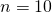andblack marbles in the population there is the potential to select anywhere between 0 and 10 black marbles.

N <- 50
n <- 10
K <- 20
k.seq <- 0:n

hypergeom <- function(N, n, K, k) exp(log(choose(K, k)) + log(choose(N-K, n-k)) - log(choose(N, n)))
k.seq <- seq(0, 10, 1)
y <- hypergeom(N, n, K, k.seq)
qplot(x = k.seq, y = y, geom = "line")The maximum likelihood occurs atmeaning on average we can expect to select 4 black marbles from the bag with a sample size of.

The second part of the problem will be simplified to,

1. The probability of selecting exactly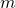matches insamples givenblack marbles are selected.

This can also be formulated as a hypergeometric distribution.The condition ofblack marbles is important since it alters the probability of getting a matching white, for example if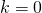marbles are selected there is a 0% chance of getting a match, similarly ifthere is a 0% chance of drawing a match. It can also be shown the maximum number of matches possible for this problem iswhich makes sense.

Combining these probabilities the joint probability of selectingblack marbles andmatches can be calculated.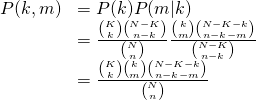In order to find the probability of selectingmatches the joint distribution needs to be summed over all possible values ofto solve for the marginal distribution.The original problem is stated as, what is the probability of selecting at least 1 match? This is similar to the birthday paradox problem. The probability of selecting at least 1 match is the same as 1 minus the probability of selecting 0 matches i.e..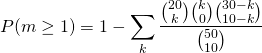prob.match <- function(N, n, K, k, m) hypergeom(N, n, K, k)*hypergeom(N-K, n-k, k, m)
p.atleast1 <- 1-sum(prob.match(N, n, K, k.seq, 0))
p.atleast1

##  0.5761024

The probability that we will selected at least 1 match is 0.5761024. Using this formulation we can also compute the expected number of matches in the sample.pm <- sapply(0:n, function(x) sum(prob.match(N, n, K, k.seq, x)))
expected.value <- sum(k.seq*pm)
expected.value

##  0.7346939

In a sample of 10 we would expect to draw (0, 1) matches. In real world problems this can help you decide on how large your sample needs to be to find specific matches in the population.

## Simulation

To check these results this scenario will be simulated 10,000 times.

# simulate matches
simulate.matches <- function(N, n, K){
pop <- 1:N
selected.sample <- sample(pop, n)
black <- sample(pop, K)
white <- sample(pop[-black], K)
black_id <- black %in% selected.sample
white_id <- white %in% selected.sample
selected.pairs <- white_id + black_id > 1 # if white and black both selected this will equal 2
return(sum(selected.pairs))
}

# run sim
draws <- replicate(1e4, simulate.matches(N, n, K))
mean(draws > 0)

##  0.5691

mean(draws)

##  0.7276

The approximate probability of selecting at least 1 match is 0.5691 and the expected number of matches is 0.7276 based on 10,000 simulations. This is very close to our analytical solution above.

## In the real world

Neat examples like this rarely occur in the real world. More often we are dealing with much larger numbers. For example, consider a case where we are required to sample the population of a country and are aiming to sample people who are hosts to a contagion. For story telling purposes person A who carries the contagion can only infect one other person, person B (not realistic but the problem is mathematically similar to something I’ve recently worked on). There are no other indicators in a structured form on the database to simply query the people in the population who carry the contagion or have received it from someone else. However by manually inspecting the persons record we are able to deduce this information. Therefore we take a sample of the population and hope to select A-B pairs.

To replicate this the parameters are set to be,

•••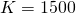The problem here is the number of ways you can a sample 200k out of 6 million records is too ridiculous to even try and quantify, it’s far beyond the number of atoms in the known universe. R will simply return NaN. In cases with such large numbers a normal approximation to the hypergeometric distribution is applied.

## Normal approximation

To make a normal approximation to the hypergeomtetric distribution the mean and standarad deviation of the hypergeometric are calculated.Since the normal distribution is continuous, to accurately approximate the probability of selectingsuccesses the mean of the probabilities evaluated at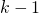and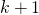. This will be tested on the smaller example first.

## normal approximation to the hypergeometric distribtuion
mu <- n*K/N
sd <- sqrt(mu*(N-K)/N*(N-n)/(N-1))
x <- seq(0, 10, 0.1)
y.norm <- dnorm(x, mu, sd)
qplot(x = x, y = y.norm, geom = "line")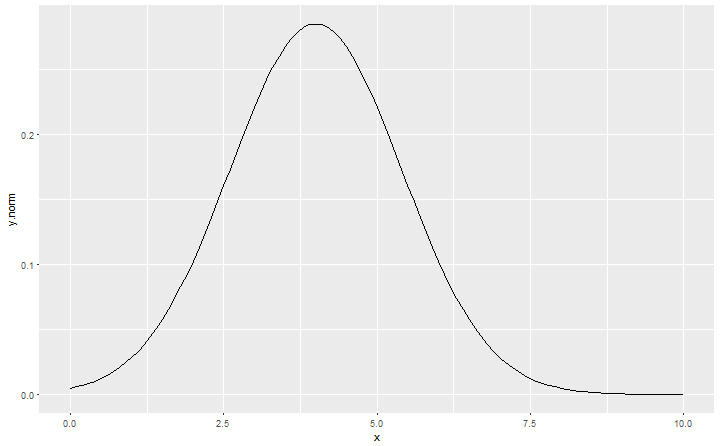hypergeom.norm <- function(N, n, K, k){
mu <- n*K/N
sd <- sqrt(mu*(N-K)/N*(N-n)/(N-1))
prob <- 1/2*(pnorm(k+1, mu, sd) - pnorm(k-1, mu, sd))
return(prob)
}

z.direct <- hypergeom(N, n, K, k.seq)
z.norm <- hypergeom.norm(N, n, K, k.seq)
df <- data.frame(k = c(k.seq, k.seq), prob = c(z.direct, z.norm), type = c(rep("direct", length(k.seq)), rep("norm approx", length(k.seq))))
ggplot(df, aes(x = k, y = prob, col = type)) + geom_line()In our example the distributions are very close. The normal approximation becomes more accurate as,andincrease.

# adapting function for normal approximation
prob.match.norm <- function(N, n, K, k, m) sum(hypergeom.norm(N, n, K, k)*hypergeom.norm(N-K, n-k, k, m))

# on the real world problem
## direct method
N <- 6e6
n <- 2e5
K <- 1500
k.seq <- seq(0, 1500, 1)

# probability at least 1
p.atleast1.norm <- 1-sum(prob.match.norm(N, n, K, k.seq, 0))
p.atleast1.norm

##  0.8564404

# expected value normal
pm.norm <- sapply(0:K, function(x) sum(prob.match.norm(N, n, K, k.seq, x)))
expected.value.norm <- sum(k.seq*pm.norm)
expected.value.norm

##  1.734464

There is an 85.6% chance of drawing at least 1 match, which isn’t particularly high when taking a sample of 200k. On average there will be between 1 and 2 matches. If you are required to detect these matched pairs in the data with a relatively low population of successes you will need to take a very large sample. It is important for situations like this to be communicated well to manage expectations. Dealing with large amounts of data can add a huge overhead to your work if the infrastructure isn’t there to support it. But knowing the odds of selecting the matched pairs you can make better decisions about how much sample to take or take more targetted samples at the beginning to speed up analysis.

## Estimating the number of successes in the population

Often you don’t know how many successes there are in the population and the goal is to estimate this number. Assume we have checked every unit in the sample and we have found 10 matches. This is considerably more than what we would have predicted when assumingand therefore necessary to re-estimate how many cases there are in the population. Given there is inherent uncertainty in the estimate it is good practice to estimate the distribution of likely values. We samplefrom the probability distribution using the grid method.

K <- seq(1000, 20000, 100)
pdist <- sapply(K, function(x) sum(prob.match.norm(N, n, x, seq(100, x, 1), 10)))
draws <- sample(K, 2e3, replace = TRUE, prob = pdist)
ggplot(data.frame(K = draws), aes(x = K)) + geom_histogram(fill = "turquoise", col = "grey20")

## stat_bin() using bins = 30. Pick better value with binwidth.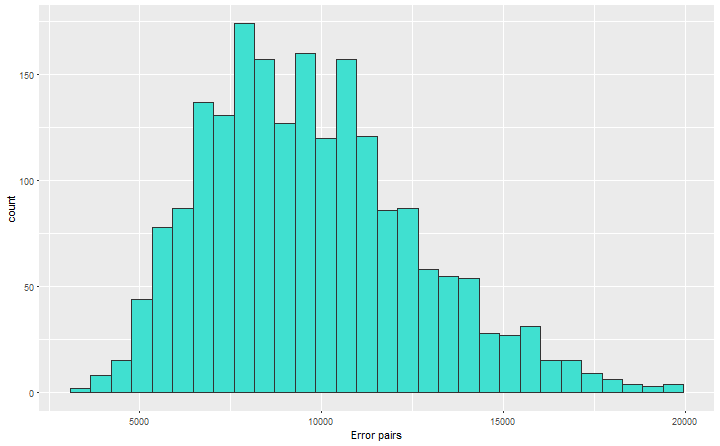q <- quantile(draws, c(0.025, 0.5, 0.975))
q

##    2.5%     50%   97.5%
##  5100.0  9400.0 16102.5

If we observed 10 matches in the sample of 200k units, it is likley there are 9400 error pairs in the population with 95% of the most likely values lying in the range (5100, 16102.5).

## Bayesian approach

The better way is to take a Bayesian approach to estimateby assuming a distribution forand(which we have done) and define a beta-binomial distribution for.Gelman et al explains the beta-binomial arises from first sampling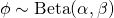and then drawing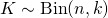. The benefit of a Bayesian approach is any prior beliefs we have about the population of carriers can be incorporated into the model. Prior information is introduced through the hyperparametersand. By setting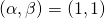we are assuming a non-informative prior and effectively get the same result as above.

Let’s assume our prior knowledge suggests it could be as high as 1 in 50 people are carriers therfore we set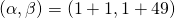. Using the metropolis-hastings algorithmcan be estimated by taking draws from the posterior.

N <- 6e6
n <- 2e5
m <- 10

K.post <- function(par){
# set pars
K <- par

# likelihood calculation
loglik <- log(prob.match.norm(N, n, K, seq(2*m, max(2*m, min(K, n)), 1), m))

# priors
K.prior <- dbeta(K/N, 2, 50, log = T)

# return posterior likelihood
return(sum(loglik, K.prior))
}

# run metropolis hastings
theta0 <- 1200
runMH <- Metro_Hastings(li_func = K.post, par = theta0, par_names = "K")

##  "updating: 10%"
##  "updating: 20%"
##  "updating: 30%"
##  "updating: 40%"
##  "updating: 50%"
##  "updating: 60%"
##  "updating: 70%"
##  "updating: 80%"
##  "updating: 90%"
##  "updating: 100%"

# plot results
df <- data.frame(iter = 1:length(runMH$trace), K.trace = runMH$trace)
grid.arrange(
ggplot(df, aes(x = K.trace)) +
geom_histogram(fill = "turquoise", col = "grey20", size = 0.1) +
geom_vline(xintercept = median(df$K.trace),lty = 2), ggplot(df, aes(x = iter, y = K.trace)) + geom_line() + geom_hline(yintercept = median(df$K.trace), col = "red", lty = 2), nrow = 1)

## stat_bin() using bins = 30. Pick better value with binwidth.# credible interval
quantile(runMH\$trace, c(0.025, 0.5, 0.975))

##      2.5%       50%     97.5%
##  5532.401 10283.820 18508.274

The influence of the prior has shifted the range of possible values ofhigher giving our best estimate of the number of carriers in the population.